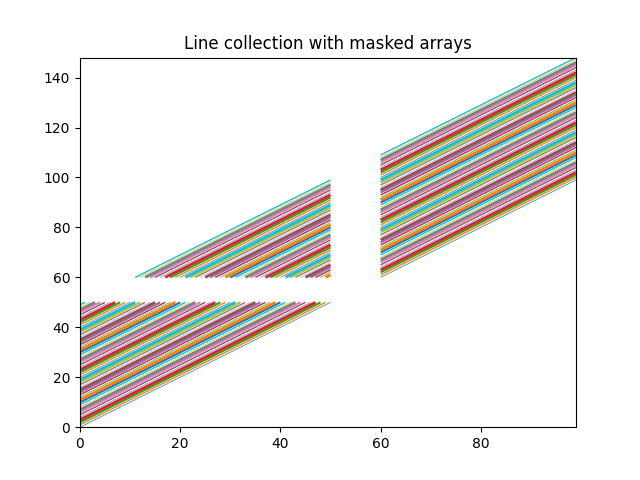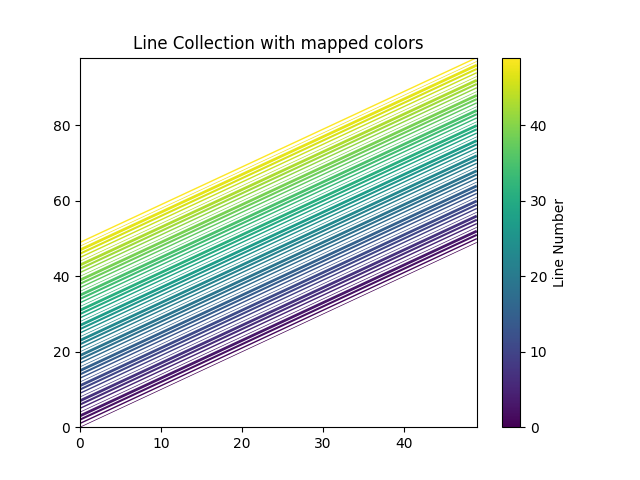# Plotting multiple lines with a LineCollection#

Matplotlib can efficiently draw multiple lines at once using a LineCollection, as showcased below.

import matplotlib.pyplot as plt
from matplotlib.collections import LineCollection

import numpy as np

x = np.arange(100)
# Here are many sets of y to plot vs. x
ys = x[:50, np.newaxis] + x[np.newaxis, :]

segs = np.zeros((50, 100, 2))
segs[:, :, 1] = ys
segs[:, :, 0] = x

segs = np.ma.masked_where((segs > 50) & (segs < 60), segs)

# We need to set the plot limits, they will not autoscale
fig, ax = plt.subplots()
ax.set_xlim(x.min(), x.max())
ax.set_ylim(ys.min(), ys.max())

# *colors* is sequence of rgba tuples.
# *linestyle* is a string or dash tuple. Legal string values are
# solid|dashed|dashdot|dotted.  The dash tuple is (offset, onoffseq) where
# onoffseq is an even length tuple of on and off ink in points.  If linestyle
# is omitted, 'solid' is used.
# See matplotlib.collections.LineCollection for more information.
colors = plt.rcParams['axes.prop_cycle'].by_key()['color']

line_segments = LineCollection(segs, linewidths=(0.5, 1, 1.5, 2),
colors=colors, linestyle='solid')
plt.show()In the following example, instead of passing a list of colors (colors=colors), we pass an array of values (array=x) that get colormapped.

N = 50
x = np.arange(N)
ys = [x + i for i in x]  # Many sets of y to plot vs. x
segs = [np.column_stack([x, y]) for y in ys]

fig, ax = plt.subplots()
ax.set_xlim(np.min(x), np.max(x))
ax.set_ylim(np.min(ys), np.max(ys))

line_segments = LineCollection(segs, array=x,
linewidths=(0.5, 1, 1.5, 2),
linestyles='solid')
axcb = fig.colorbar(line_segments)
axcb.set_label('Line Number')
ax.set_title('Line Collection with mapped colors')
plt.sci(line_segments)  # This allows interactive changing of the colormap.
plt.show()References

The use of the following functions, methods, classes and modules is shown in this example:

Total running time of the script: ( 0 minutes 1.020 seconds)

Gallery generated by Sphinx-Gallery